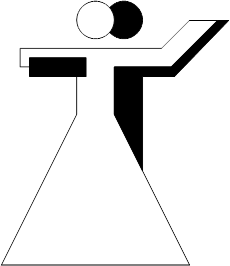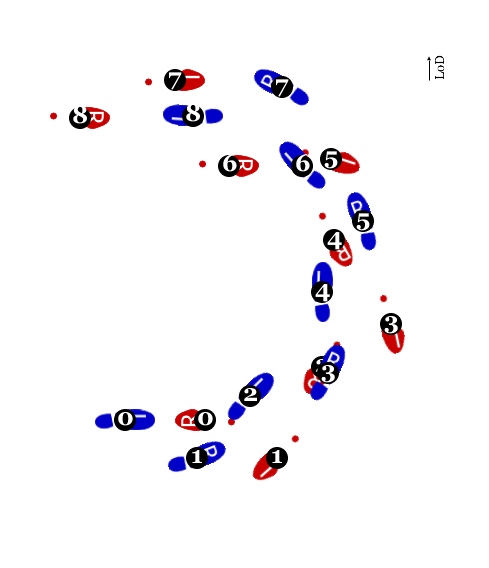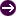# Basic movementen [de]

Eight small steps forward (backward for the lady) in a half circle to the left## Steps (man)

0 Begin in closed hold, facing the wall, weight on the LF.
1 Take a step forward (RF). [slow]
RF in place. Make an eight turn to the left.
2 Take a step forward (LF). [slow]
LF in place. Make an eight turn to the left.
3 Take a step forward (RF). [slow]
RF in place. Make an eight turn to the left.
4 Take a step forward (LF). [slow]
LF in place. Make an eight turn to the left.
5 Take a step forward (RF). [slow]
RF in place. Make an eight turn to the left.
6 Take a step forward (LF). [slow]
LF in place. Make an eight turn to the left.
7 Take a step forward (RF). [slow]
RF in place. Make an eight turn to the left.
8 Take a step diagonally forward and to the left (LF). [slow]
LF in place. Make an eight turn to the left.

0 Begin in closed hold, facing the centre, weight on the RF.
1 Take a step backward (LF). [slow]
LF in place. Make an eight turn to the left.
2 Take a step backward (RF). [slow]
RF in place.
3 Take a step backward (LF). [slow]
LF in place. Make an eight turn to the left.
4 Take a step backward (RF). [slow]
RF in place.
5 Take a step backward (LF). [slow]
LF in place. Make an eight turn to the left.
6 Take a step backward (RF). [slow]
RF in place.
7 Take a step diagonally backward and to the left (LF). [slow]
LF in place.
8 Take a step backward (RF). [slow]
RF in place.

## Preceding figuresSur place

## Following figuresSur place

Verbal and animated descriptions of latin and ballroom dancing figures